;nbsp

Hi parents and students! This is an introduction to what you’ll be needing to know by the end of the first Geometry 2 semester. My courses cover all of the common core requirements (the syllabus for semester 1 is here, and semester 2 is here!) and prepare my students to think critically. Not only do I want your student to know the material in my class, I want them to succeed in all future classes and opportunities. Please try out this sample test to see what your child will be learning this semester!

Name*

This is a required question

Email*

Must be a valid email address
This is a required question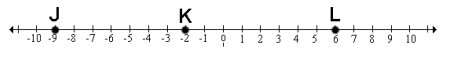1. What is the length of LK?
This is a required question

2. Find the midpoint of JL
This is a required question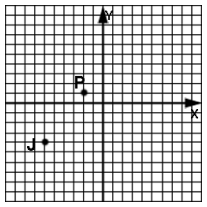3. J is the midpoint of PK (K not pictured). What are the coordinates of K?
This is a required question

4. Use the points given in problem 12 to find the midpoint of line segment PJ.
This is a required question

5. If twice the measure of an angle is 6 more than its complement, what is the measure of the angle?
Set up an equation with (x) and (90-x)! Solve for x.
This is a required question

## Use the following drawing for problems 6 and 7.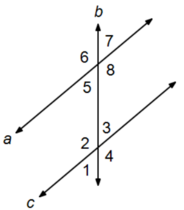6. If m∠5 = (5x – 15)° and m∠3 = (3x + 15)°, find x.
This is a required question

7. If m∠4 = 140°, find m∠7.
This is a required question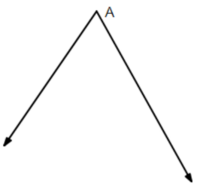8. Identify the bisector of angle A.
This is a required question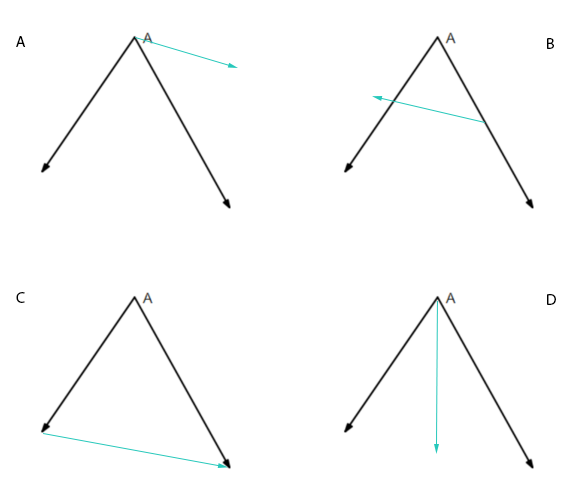## Use this shape for problems 9 and 10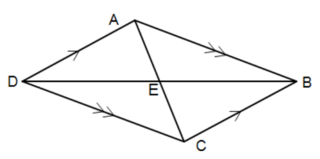9. If m∠DAB = 120°, what is m∠ADC?
This is a required question

10. If DC = 40, what is the length of AB?
This is a required question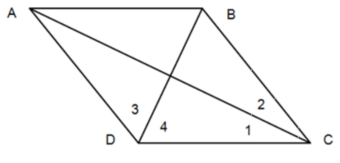11. In rhombus ABCD m∠4 = (25x)° and m∠1 = (10x – 5)°. Find x.
This is a required question

## Use this shape for problems 12 and 13.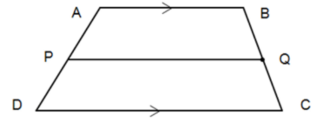12. If AB = (4x – 10), DC = (3x + 8), and the median has a length of 13, find x.
This is a required question

13. If AB = 10 and PQ = 13.5, what is DC?
This is a required question

14. Identify the 30-60-90 triangle that has labeled sides with the proper “standard lengths”
This is a required question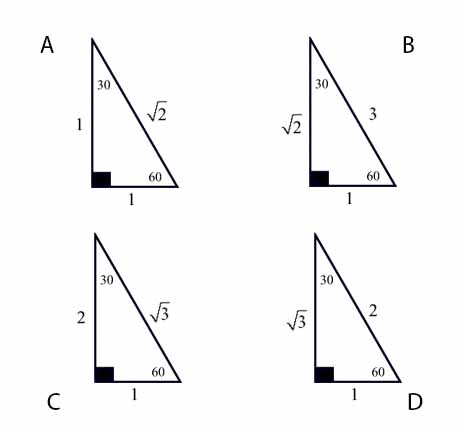15. Identify the 45-45-90 triangle that has labeled sides with the proper “standard lengths”
This is a required question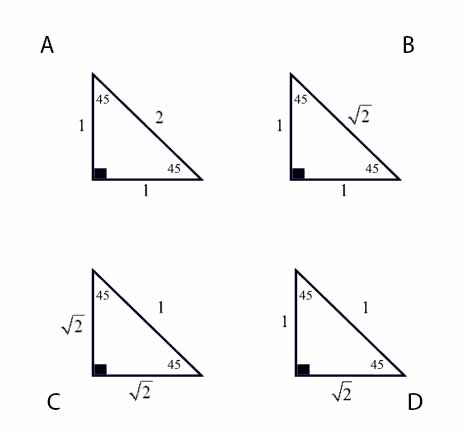16a. Define sine in terms of opp, adj, and hyp.
This is a required question

16b. Define cos in terms of opp, adj, and hyp.
This is a required question

16c. Define tan in terms of opp, adj, and hyp.
This is a required question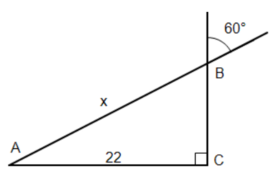17. Using the ratios of a special triangle, find x.
This is a required question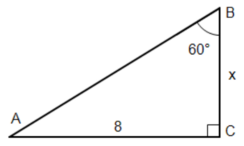18. Using the ratios of a special triangle, find x.
This is a required question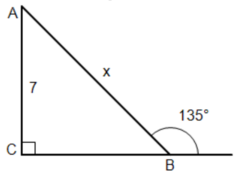19. Using the ratios of a special triangle, find x.
This is a required question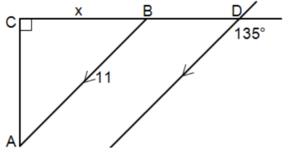20. Using the ratios of a special triangle, find x.
This is a required question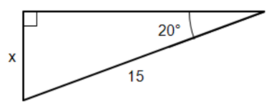21. Using the trig functions, find x.
This is a required question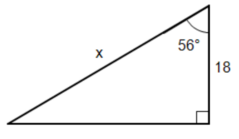22. Using the trig functions, find x.
This is a required question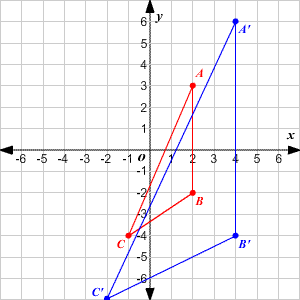23. A’B’C’ is a dilation of ABC about the origin. What is the scale factor?
This is a required question

24. A monkey whose head is only 3′ off the ground looks at a mirror on the ground that is 6′ in front of him. He sees a banana in a tree that is another 20′ on the other side of the mirror. How high is the banana?
Proportional triangles. Solve for x.
This is a required question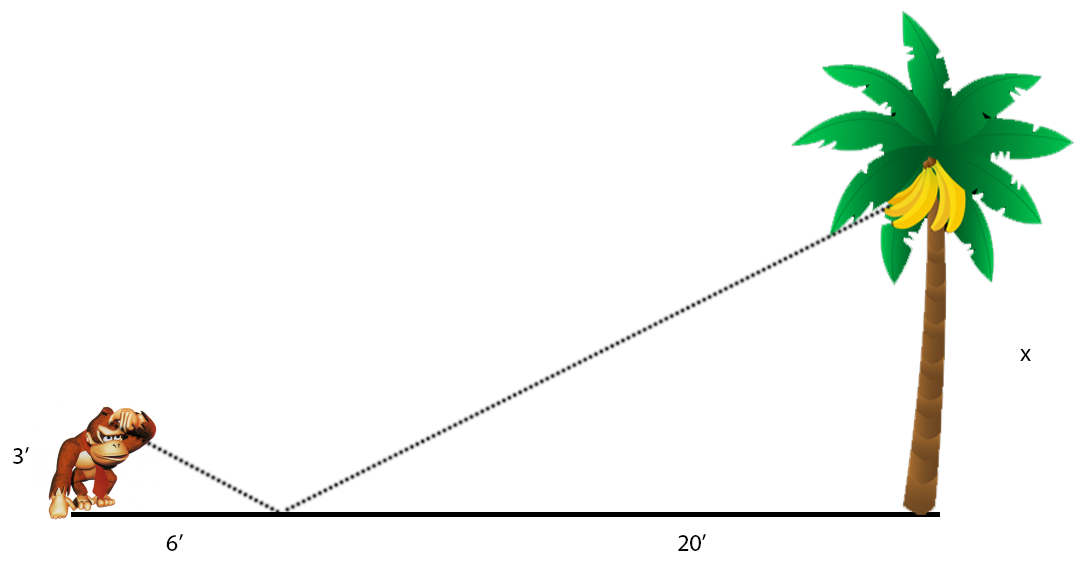25. Three cities (Culver, Preston, and Abbot) form a triangle on a map. On the map, Preston is 3.25” from Abbot while Abbot is 2” from Culver. If the actual distance from Abbot to Culver is 186 miles, how far is it from Preston to Abbot?
Proportional triangles. Solve for x.
This is a required question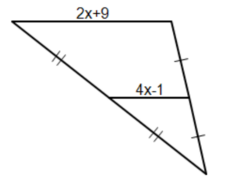26. Find x.
This is a required question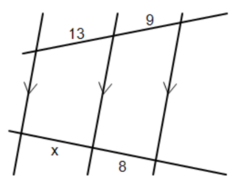27. Find x.
This is a required question28. Find x if AB = 60.
Cut AB into 3 sections of equal length!
This is a required question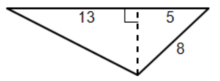29. Find the area of this triangle.
This is a required question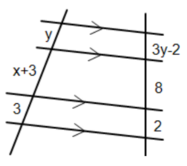30a. Find x.
This is a required question

30b. Find y.
This is a required question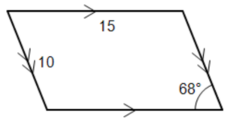31a. Find the perimeter of this parallelogram.
This is a required question

31b. Find the area of this parallelogram.
This is a required question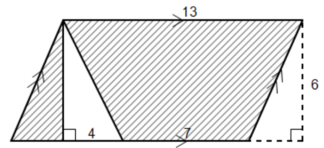Find the area of both the parallelogram and the triangle to help find the shaded area.
This is a required question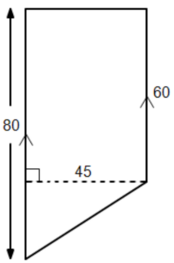33. Find the area of this trapezoid.
This is a required question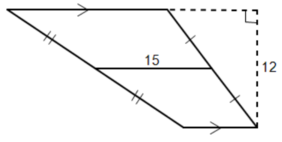34. Find the area of this trapezoid.
This is a required question

This is a required question